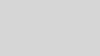# Testing large quantum algorithms using sparse simulationMathias Soeken

We are excited to announce that the sparse simulator has been shipped with the newest release of the QDK 0.24.201332. Sparse simulation achieves orders of magnitude reductions in memory requirements and runtime for a wide range of applications and allows you to simulate some quantum algorithms with more than 100 qubits.

### How it works

The sparse simulator (`SparseSimulator`) makes use of the fact that, for many applications, the quantum state has relatively few nonzero amplitudes. Instead of storing these zeros, which is what the full state simulator (`QuantumSimulator`) does, the sparse simulator only stores nonzero amplitudes together with an index identifying the corresponding computational basis state.The main sources of sparsity in the quantum state are (1) structure in the underlying problem such as particle conservation in physical systems and (2) the use of auxiliary qubits in, e.g., arithmetic subroutines such as modular exponentiation in Shor’s algorithm for factoring. For (2), note that these auxiliary qubits don’t increase the number of nonzero amplitudes in the superposition, but just how many bits are in each computational basis state.

In addition to only storing nonzero amplitudes, the sparse simulator has a built-in mechanism to queue certain quantum gates to keep the state sparse for as long as possible. One example of quantum operations that are queued are Hadamard gates: These are only executed once it is no longer possible to easily commute incoming quantum operations through the queued Hadamard gates. As a simple example, consider two qubits with a Hadamard gate on each qubit, followed by a controlled NOT (CNOT) gate. Instead of directly applying the Hadamard gates (which could increase the number of nonzero amplitudes in the state vector), we can commute the CNOT through them, and apply a CNOT with flipped control and target qubit, keeping the two Hadamard gates in our queue of gates that are yet to be applied. The following circuit diagrams illustrate this mechanism:### Full state simulator vs. sparse simulator

Let’s see how the two simulators perform when simulating Shor’s algorithm for determining the prime factors p and q of N = p · q. The sample can be found in the Q# samples repository.

When using Toffoli-based arithmetic, the sparse simulator clearly outperforms the full-state simulator, which only managed to simulate the smallest instance within 1 minute. With the sparse simulator, on the other hand, we can even simulate factoring N=1443, which used 49 qubits!Even when the state vector is not particularly sparse, the sparse simulator often outperforms the highly optimized and multithreaded full state simulator. One example is Shor’s algorithm with Fourier-based arithmetic. Compared to the Toffoli-based implementation, there are fewer auxiliary qubits in the implementation and the quantum Fourier transforms make the states denser, both of which reduce the runtime advantage of the sparse simulator. However, the advantage is still substantial at larger problem sizes even if it uses only a single core, whereas the full state simulator uses all four cores.All of these benchmarks were run on a ThinkPad X1 laptop with four cores and we report the median runtime of `dotnet run --no-build -- [application arguments]` out of three runs. For reproducibility, we manually set the generator to two in `Shor.qs` (instead of selecting it at random).